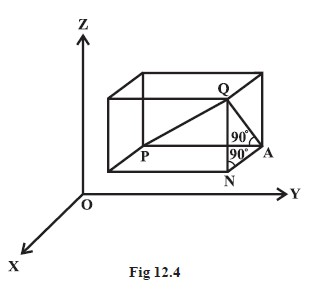Mathematics Distance between Two Points
Click for Only Video

### Topic Covered

star Distance between Two Points

### Distance between Two Points\color{red} ✍️ We have studied about the distance between two points in two-dimensional coordinate system. Let us now extend this study to three-dimensional system.

\color{red} ✍️ Let P(x_1, y_1, z_1) and Q ( x_2, y_2, z_2) be two points referred to a system of rectangular axes OX, OY and OZ.

\color{red} ✍️ Through the points P and Q draw planes parallel to the coordinate planes so as to form a rectangular parallelopiped with one diagonal PQ (Fig 12.4).

Now, since ∠PAQ is a right angle, it follows that, in triangle PAQ,

color(blue)(PQ^2 = PA^2 + AQ^2) ................................ (1)

Also, triangle ANQ is right angle triangle with ∠ANQ a right angle.

Therefore color(blue)(AQ^2 = AN^2 + NQ^2) ................................. (2)

From (1) and (2), we have

 \ \ \ \ \ \ \ \ \ \ \ \ \ \ \ \ \ \ color(green)(PQ^2 = PA^2 + AN^2 + NQ^2)

Now PA = y_2 – y_1,  \ \ AN = x_2 – x_1 and NQ = z_2 – z_1

Hence PQ^2 = (x_2 – x_1)^2 + (y_2 – y_1)^2 + (z_2 – z_1)^2

Therefore color{red}(PQ = sqrt ((x_2 – x_1)^2 + (y_2 – y_1)^2 + (z_2 – z_1)^2))

This gives us the distance between two points (x_1, y_1, z_1) and (x_2, y_2, z_2).

\color{red} ✍️ In particular, if color(blue)(x_1 = y_1 = z_1 = 0), i.e., point P is origin O,

then color{orange}(OQ = sqrt( x_2^2 +y_2^2 +z_2^2) ,) which gives the distance between the origin O and any point Q (x_2, y_2, z_2).
Q 3059634514Find the distance between the points P(1, –3, 4) and Q (– 4, 1, 2).Solution:

The distance PQ between the points P (1,–3, 4) and Q (– 4, 1, 2) is

PQ = sqrt((-4-1)^2+(1+3)^2+(2-4)^2)

= sqrt(25+16+4)

= sqrt(45) =3 sqrt3 units
Q 3089834717Are the points A (3, 6, 9), B (10, 20, 30) and C (25, – 41, 5), the vertices of a right angled triangle?Solution:

By the distance formula, we have
AB^2 = (10 – 3)^2 + (20 – 6)^2 + (30 – 9)^2

= 49 + 196 + 441 = 686

BC^2 = (25 – 10)^2 + (– 41 – 20)^2 + (5 – 30)^2

= 225 + 3721 + 625 = 4571
CA^2 = (3 – 25)^2 + (6 + 41)^2 + (9 – 5)^2
= 484 + 2209 + 16 = 2709
We find that CA^2 + AB^2 ≠ BC^2.
Hence, the triangle ABC is not a right angled triangle.
Q 3019034810Find the equation of set of points P such that PA^2 + PB^2 = 2k^2, where A and B are the points (3, 4, 5) and (–1, 3, –7), respectively.Solution:

Let the coordinates of point P be (x, y, z).
Here PA^2 = (x – 3)^2 + (y – 4)^2 + ( z – 5)^2
PB^2 = (x + 1)^2 + (y – 3)^2 + (z + 7)^2
By the given condition PA^2 + PB^2 = 2k^2, we have
(x – 3)^2 + (y – 4)^2 + (z – 5)^2 + (x + 1)^2 + (y – 3)^2 + (z + 7)^2 = 2k^2
i.e., 2x^2 + 2y^2 + 2z^2 – 4x – 14y + 4z = 2k^2 – 109.